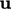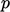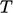## Chapter 8Sample Problems

“I know there’s an answer, I know now but I have to ﬁnd it by myself.”

The Beach Boys, I Know There’s An Answer (1966).

The preceding chapters establish some general principles of CFD, covering topics in ﬂuid dynamics, numerical methods, boundary conditions, algorithms, solvers and turbulence and its modelling.

This chapter aims to bring together some of these principles using four sample CFD problems, some of which are mentioned in the earlier chapters. The four examples demonstrate a variety of ﬂow types, including:

• two internal ﬂows, inside a room and a Venturi tube, in which, beyond regions of signiﬁcant inﬂow and outﬂow, the ﬂuid is contained by solid boundaries;
• two external ﬂows, around a vehicle and a cylinder, in which a considerable part of the domain is unbounded.

The ﬂow in the room and around the vehicle are turbulent, so demonstrate turbulence modelling and wall functions from Chapter 7 . The ﬂow is laminar in the other examples.

The vehicle and Venturi tube cases are simulated using the steady-state algorithm in Sec. 5.12 , whereas the room and cylinder cases use the transient algorithm in in Sec. 5.19 .

### Mesh generation

The sample problems are supported by additional information on mesh generation. Strategies for meshing between the far-ﬁeld and a body of interest in external ﬂows are discussed in Sec. 8.1 .

Meshes may include cells with high aspect ratio to increase the solution eﬃciency, particularly with external ﬂows. Including these cells, however, increases the range of magnitudes of the matrix coeﬃcients, which adversely aﬀects solution convergence, as discussed in Sec. 8.2 .

The generation of block-structured and unstructured meshes are discussed in Sec. 8.3 and Sec. 8.5 respectively. The block-structured approach is suited to problems whose boundaries are described by relatively simple geometric forms, e.g. cylinders, cuboids, etc.

The unstructured approach is designed to accommodate more complex boundary geometries, e.g. the vehicle in the external aerodynamics example. The geometries are typically deﬁned by a computer-aided design (CAD) model.

### Boundary Conditions

The examples demonstrate a variety of specialised boundary conditions. The cylinder and vehicle both use the freestream condition, described in Sec. 4.16 at the far-ﬁeld boundary. The Venturi example uses a nonuniform inlet condition for, described in Sec. 8.7 . Finally, the ﬂow in a room uses a condition forto ensure correct boundary ﬂuxes, as described in Sec. 5.11 and the external heat ﬂux condition forfrom Sec. 4.17 .
Notes on CFD: General Principles - Chapter 8 Sample Problems